# JEE Main Friction Previous Year Questions with Solutions

When a ball is rolled on a surface it comes to rest after a while due to frictional force. The resistance offered by the surface on the rolling ball is called friction. The direction of friction is opposite the direction of motion of the ball. There are different types of friction like Static Friction, Sliding Friction, Rolling Friction and Fluid Friction. The frictional force is affected by the angle and position of the object, weight of the object and much more.

All the important questions from the topic “Friction” from the previous years’ JEE question papers are listed out in this page. All the IIT JEE aspirants are advised to download the past year questions and start practising to score good marks in JEE.

## JEE Main Previous Year Solved Questions on Friction

Q1: During peddling of a bicycle, the force of friction exerted by the ground on the two wheels is such that it acts

(a) in the backward direction on the front wheel and in the forward direction on the rear wheel

(b) in the forward direction on the front wheel and in the backward direction on the rear wheel

(c) in the backward direction on both, the front and the rear wheels

(d) in the forward direction on both, the front and the rear wheels.

Answer: (a) Due to peddling, the point of contact of the rear wheel has a tendency to move backwards. So frictional force opposes the backwards tendency i.e., the frictional force acts in the forward direction. But the back wheel accelerates the front wheel in the forward direction. To oppose this frictional force acts in the backward direction on the front wheel.

Q2: A block of mass 0.1 kg is held against a wall applying a horizontal force of 5N on the block. If the coefficient of friction between the block and the wall is 0.5, the magnitude of the frictional force acting on the block is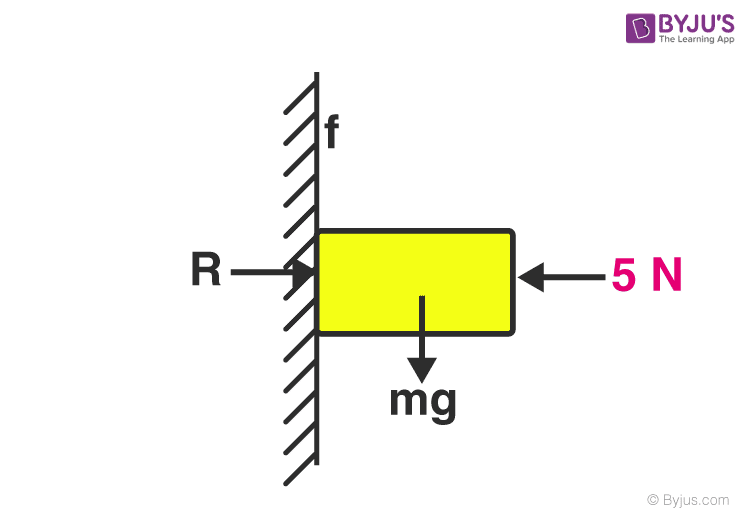(a) 2.5N

(b) 0.98N

(c) 4.9N

(d) 0.49N

Solution

Consider the forces, acting on the block in the vertical direction

Force of friction f = μR

f= 0.5 X 5

f = 2.5 N

Q3: A horizontal force of 10 N is necessary to just hold a block stationary against a wall. The coefficient of friction between the block and the wall is 0.2. The weight of the block is-

(a) 20 N

(b) 50 N

(c) 100 N

(d) 2 N

Solution

Frictional force balances the weight of the body

Frictional force f = μN = mg

f= 0.2 X 10 = 2N

Therefore, weight of the block mg= 2N

Q4: A marble block of mass 2 kg lying on ice when given a velocity of 6 m/s is stopped by friction in 10s. Then the coefficient of friction is (consider g =10m/s)

(a) 0.02

(b) 0.03

(c) 0.06

(d) 0.01

Solution

u = 6 m/s

v = 0

t =10s

a = -f/m = – μmg/m = – μg = -10μ

Substituting values in v = u+at

0 = 6 – 10μ x 10

Therefore, μ = 0.06

Q5: A block P of mass m is placed on a horizontal frictionless plane. The second block of the same mass m is placed on it and is connected to a spring of spring constant k. The two blocks are pulled by a distance A. Block Q oscillates without slipping. What is the maximum value of the frictional force between the two bloc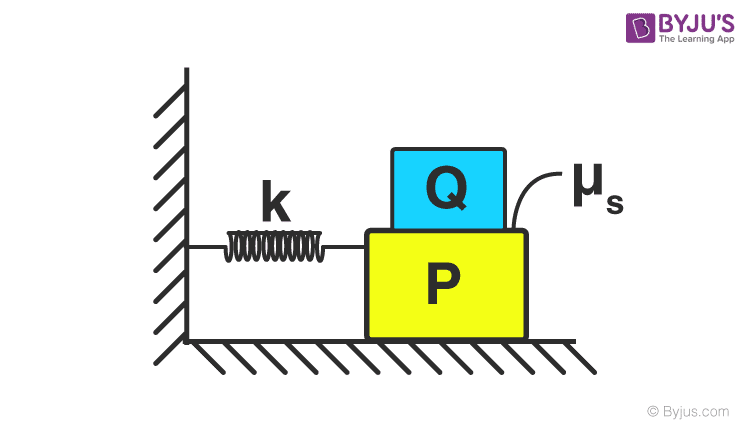(a)kA/2

(b) kA

(c) μsmg

(d) zero

Solution

Block Q oscillates but does not slip on P. It means that acceleration is the same for Q and P both. There is a force of friction between the two blocks while the horizontal plane is frictionless. The spring is connected to the upper block. The (P – Q) system oscillates with angular frequency ω. The spring is stretched by A.

Therefore, $\omega = \sqrt{k/2m}$

Therefore, Maximum acceleration in SHM = ω2A

am = kA/2m

Now consider the lower block.

Let the maximum force of friction = fm

fm= mam or fm= m x kA/2m

fm= kA/2

Q6: A block of mass m starts moving on a rough horizontal surface with a velocity v. It stops due to friction between the block and the surface after moving through a certain distance. The surface is now tilted to an angle of 30°with the horizontal and the same block is made to go up on the surface with the same initial velocity v. The decrease in mechanical energy in the second situation is smaller than that in the first situation.

Statement-2: The coefficient of friction between the block and the surface decreases with the increase in the angle of inclination.

(a) Statement-1 and 2 are true and statement-2 is a correct explanation for statement-1

(b) Statement-1 and 2 are true and statement-2 is not a correct explanation for statement-1

(c) Statement-1 is true, statement-2 is false

(d) Statement-1 is false, statement-2 is true.

Answer: (c) Statement-1 is true, statement-2 is false

Q7: A block rests on a rough inclined plane making an angle of 300 with the horizontal. The coefficient of static friction between the block and the plane is 0.8. If the frictional force on the block is 10 N, the mass of the block (in kg) is : (taken g =10 m/s2)

(a) 2.0

(b) 4.0

(c) 1.6

(d) 2.5

Solution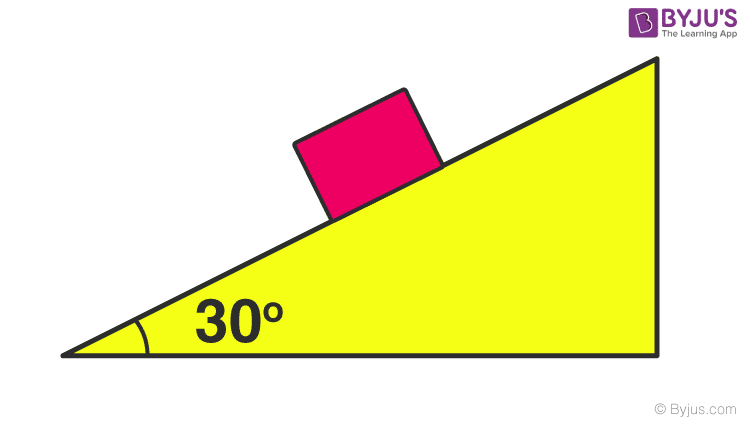μ = 0.8

f = 10 N

mg sin 30 = 10

m x 10 x (½) =10

m = 2kg

Q8. A smooth block is released at rest on a 450 incline and then slides a distance d. The time taken to slide is n times as much to slide on a rough incline than on a smooth incline. The coefficient of friction is-

(a) $\mu k=1- \frac{1}{n^{2}}$

(b) $\mu k=\sqrt{1- \frac{1}{n^{2}}}$

(c) $\mu s=1- \frac{1}{n^{2}}$

(d) $\mu s=\sqrt{1- \frac{1}{n^{2}}}$

Solution

On a smooth plane

d = ½ (g sinθ) t1

$t_{1}=\sqrt{\frac{2d}{gsin\theta }}$

On a rough surface

d = ½ (g sinθ – μg cosθ) t2

$t_{2}=\sqrt{\frac{2d}{g sin\theta- \mu g cos\theta }}$

From the question, we know

t2=nt1

$\sqrt{\frac{2d}{g sin\theta- \mu g cos\theta }}$ = $n\sqrt{\frac{2d}{g sin\theta}}$ $n=\frac{1}{\sqrt{1- \mu _{k}}}$ (since cos 450 = sin 450 $\frac{1}{\sqrt{2}}$)

$n^{2}=\frac{1}{{1- \mu _{k}}}$

1-μk = 1/n2

μk=1- 1/n2

Q9: The upper half of an inclined plane with inclination Φ is perfectly smooth, while the lower half is rough. A body starting from rest at the top will again come to rest at the bottom, if the coefficient of friction for the lower half is given by

(a) 2 sinΦ

(b) 2 cosΦ

(c) 2 tanΦ

(d) tanΦ

Solution

For the upper half of the inclined plane

v2=2a1s

Where a1 = g sinΦ

For the lower half of the inclined plane

0 = v2 – 2a2 s

Where a2 = μgcosΦ- g sinΦ

From the above equations

g sinΦ = μgcosΦ- g sinΦ

2g sinΦ = μgcosΦ

2 tanΦ = μ

Q10: Consider a car moving on a straight road with a speed of 100 m/s. The distance at which a car can be stopped is : (μk = 0.5)

(a) 800 m

(b) 1000 m

(c) 100 m

(d) 400 m

Solution

When the car is stopped by friction then its retarding force is

ma = μR

ma = μmg

a= μg

Consider the equation v2 = u2 -2as (the car is retarding so a is negative)

0 = u2 -2as

2as = u2

s = u2/2a

s = u2/2μg

s = (100)2/2 x 0.5 x 10

s = 1000 m

Q11: The minimum force required to start pushing a body up a rough (frictional coefficient μ) inclined plane F1 while the minimum force needed to prevent it from sliding down is F2. If the inclined plane makes an angle θ from the horizontal such that tan θ= 2μ then the ratio F1/F2 is

(a) 4

(b) 1

(c) 2

(d) 3

Solution

We have to work against the sliding force due to gravity and frictional force to push upwards. Therefore, the force F1= mgsinθ + μmgcosθ.

To stop the body from sliding work is done against sliding force but frictional force stops the body from sliding.

Therefore, the force F2= mgsinθ – μmgcosθ.

F1/F2 = mgsinθ + μmgcosθ/ mgsinθ – μmgcosθ

F1/F2 = tanθ +μ / tanθ – μ

(since tanθ = 2μ)

F1/F2 =2μ +μ /2μ – μ

F1/F2 = 3

Q12: A body of mass m=10−2 kg is moving in a medium and experiences a frictional force F=−kv2. Its initial speed is v0= 10 ms−1. If, after 10 s, its energy is ⅛ mv02, the value of k will be

(a) 10−3 kg m−1

(b) 10−3 kg s−1

(c) 10−4 kg m−1

(d) 10−1 kg m−1 s−1

Solution

Mass m = 102 Kg

Initial velocity v0= 10 ms−1

Time t = 10 s

Frictional Force F=− kv2

1/2 mvf2 = ⅛ mv02

vf = v0 /2

vf = 10 /2 = 5 m/s

Now, the force is

(10-2)dv/dt = -kv2

$\int_{10}^{5}\frac{dv}{v^{2}}=-100k\int_{0}^{10}dt$

⅕ = 1/10 = 100k x 10

k = 10-4

Q13: A block of mass m is placed on a surface with a vertical cross-section given by y =x3/ 6. If the coefficient of friction is 0.5, the maximum height above the ground at which the block can be placed without slipping is

(a) ⅓ m

(b) ½ m

(c) ⅙ m

(d) ⅔ m

Solution

For equilibrium under limiting friction

mg sinθ = μmgcosθ

tanθ = μ———(1)

The equation of the surface is given by y = x3/ 6

Slope dy/dx = x2/ 2——-(2)

From (1) and (2) we get

μ = x2/ 2 = 0.5

x= 1

So, y =⅙

Q14: Given in the figure are two blocks A and B of weight 20 N and 100 N, respectively. These are being pressed against a wall by a force F as shown. If the coefficient of friction between the blocks is 0.1 and between block B and the wall is 0.15, the frictional force applied by the wall on block B is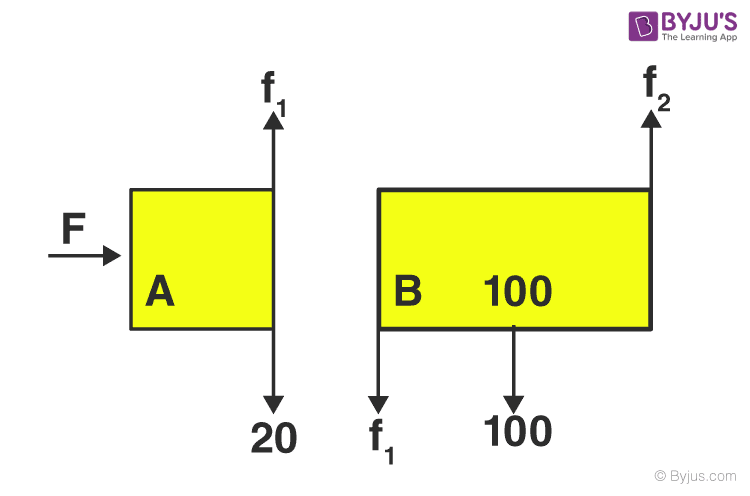(a) 120 N

(b) 150 N

(c) 100 N

(d) 80 N

Solution

For equilibrium of A

f1 = 20

For equilibrium of B

f2 = f1 + 100

f2 = 120 N

Q15: Two masses m1= 5 kg and m2= 10 kg, connected by an inextensible string over a frictionless pulley, are moving as shown in the figure. The coefficient of friction of the horizontal surface is 0.15. The minimum weight m that should be put on top of m2 to stop the motion is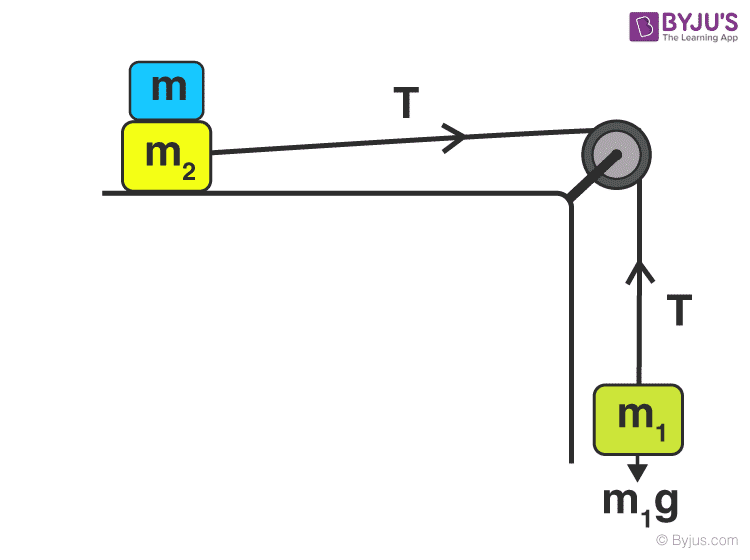(a) 27.3 kg

(b) 43.3 kg

(c) 10.3 kg

(d) 18.3 kg

Solution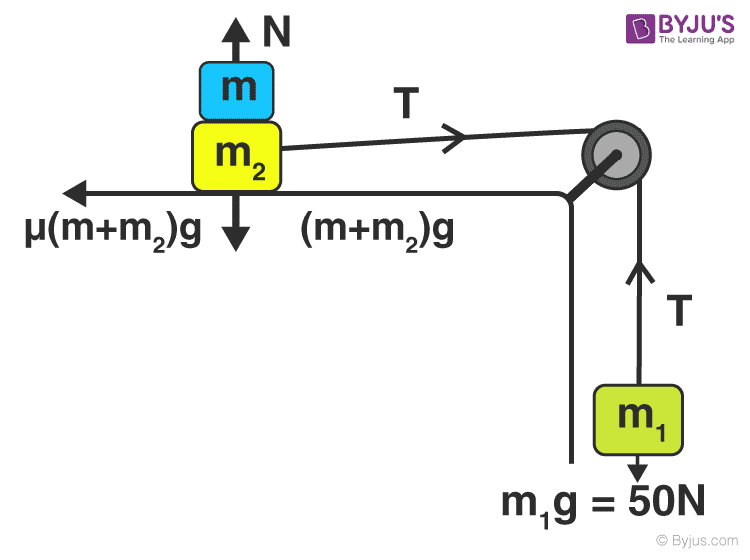50 – T = 5 × a

T – 0.15 (m + 10) g = (10 + m)a

a = 0 for rest

50 = 0.15 (m + 10) 10

5 =3/20(m + 10)

100/3= m + 10 m = 23.3 kg

So the minimum weight required from the options given is 27.3 kg